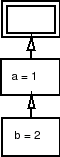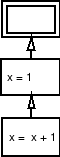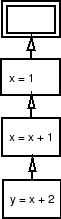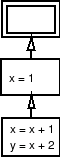# CS 312 Recitation 21 Let Expressions & the Environment Model

## Let-Expressions

When any single SML let-expression has multiple declarations in it, the declarations are evaluated and bound sequentially. This means that the following pairs of code are essentially equivalent:
 Example Expression Equivalent Expression Diagram Example #1 ```let val a = 1 val b = 2 in a + b end ``` ```let val a = 1 in let val b = 2 in a + b end end ```Example #2 ```let val x = 1 val x = x + 1 in x + x end ``` ```let val x = 1 in let val x = x + 1 in x + x end end ```Example #3 ```let val x = 1 in let val x = x + 1 val y = x + 2 in x + y end end ``` ```let val x = 1 in let val x = x + 1 in let val y = x + 2 in x + y end end end ```## Parallel Let-Expressions

One can image another language in which the multiple declarations in a let-expression are not bound sequentially, but instead in parallel. In this case, declarations can not refer to other (previous) bindings in the same let-block, but only identifiers which were bound before entering the let-block. With these semantics, the bindings may be evaluated in parallel.

Notice that this means that example 2 is an invalid expressions in this model, because x is unbound in the second declaration.

This means that in the last example, the line which states val y = x + 2 does not refer to the binding of x from the previous line, but instead the binding of x which existed before entering the let block. With the regular SML-semantics, the last example evaluates to 6, while with the other semantics this block evaluates to 5.

Below is the the environment model which results from evaluating the last example in this modified language.Contrast this environment diagram with the environment diagram presented under regular SML rules.